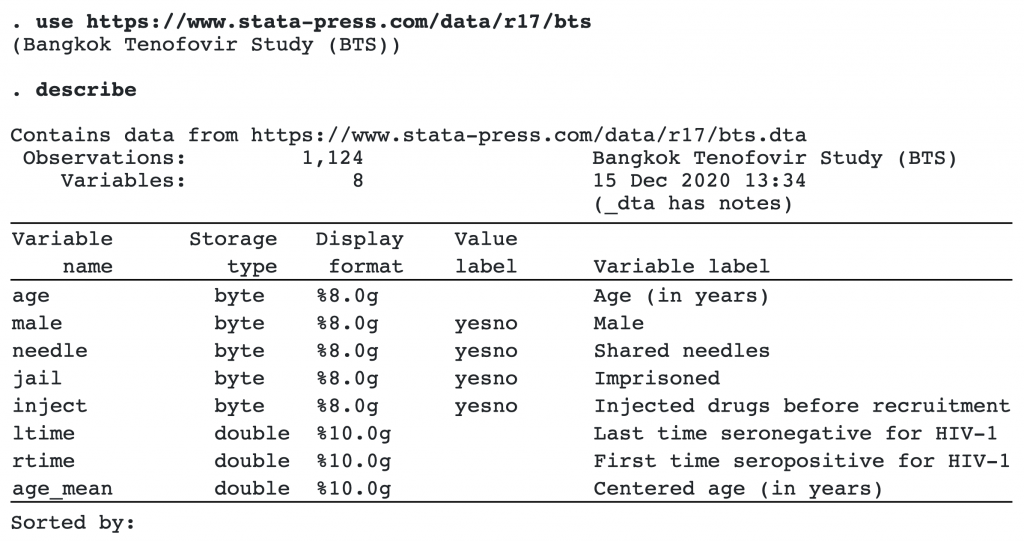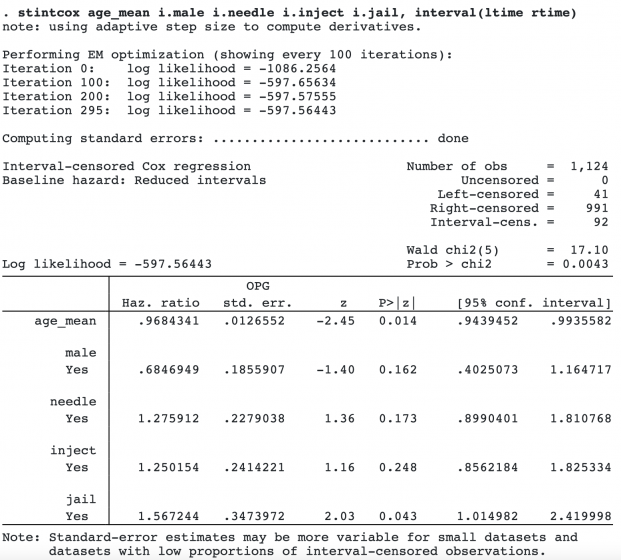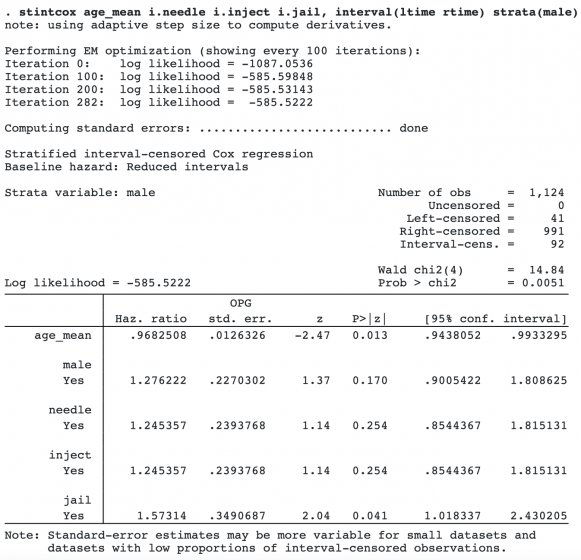## Interval-censored Cox model

What is the exact time of cancer recurrence? Or time of COVID-19 infection? We don’t really know exactly. All we know is that the cancer recurred sometime between two follow-up examinations and that the infection occurred sometime before the onset of symptomps or a positive COVID test. These are examples of interval-censored and left-censored time-to-event data. By time-to-event or event-time data, we also mean failure-time data, duration data, and so on.

Interval-censored event-time data arise in many areas, including medical, epidemiological, economic, financial, and sociological studies. Ignoring interval-censoring will often lead to biased estimates.

A semiparametric Cox proportional hazards regression model is used routinely to analyze uncensored and right-censored event-time data. In Stata 17, you can use the new estimation command stintcox to fit the Cox model to interval-censored event-time data.

Just as with right-censored data, a Cox model is appealing for interval-censored data because it uses semiparametric estimation and thus does not require any parametric assumptions about the baseline hazard function. Also for low-event rates, the exponentiated regression parameters approximate the log relative-risks.

Semiparametric estimation of interval-censored event-time data is challenging because none of the event times are observed exactly. Thus, “semiparametric” modeling of these data often resorted to using spline methods or piecewise-exponential models for the baseline hazard function. Genuine semiparametric modeling of interval-censored event-time data was not available until recent methodological advances, which are implemented in the stintcox command.

## Let’s see it work

Estimation

Graphing survivor functions

Stratification

Checking proportional-hazards assumption

## Estimation

We use data from Zeng, Mao, and Lin (2016), who study time to HIV infection in a cohort study of injecting drug users in Thailand. The dataset contains 1,124 subjects. Those subjects initially tested negative for the HIV virus. They were then followed and assessed for HIV-1 seropositivity through blood tests approximately every four months. Because the subjects were tested periodically, the exact time of HIV infection was not observed, but it was known to fall in intervals between blood tests with the lower and upper endpoints recorded in variables ltime and rtime.

We want to identify the factors that influence time to HIV infection. The factors we are interested in are age at recruitment (age), sex (male), history of needle sharing (needle), history of drug injection (inject), and whether a subject has been in jail at the time of recruitment (jail).We fit a Cox proportional hazards model in which time to HIV infection depends on the above factors of interest. To make the interpretation of the baseline hazard function more reasonable, we will use the centered age variable, age_mean.We find that age at recruitment is associated with lower risk of HIV infection and being in jail at enrollment is associated with higher risk of HIV infection. Other factors are not statistically significant.

## Graphing survivor functions

We can graph the baseline survival curve by using stcurve with all covariates set to 0.

```. stcurve, survival at((zero) _all)
```We can also graph the survivor functions with covariates set to any value. Suppose we want to compare the survivor functions of an average subject in the female and male groups. We type

```. stcurve, survival at(male=(0 1))
```## Stratification

If we assume that the baseline hazard function for female is different from that for male, we can fit a stratified Cox proportional hazards model by using the strata() option:Our conclusion here is similar to the case without stratification.

## Checking proportional-hazards assumption

It is important to evaluate the validity of the underlying assumption for the Cox proportional hazards model, which is that the hazard ratio is constant over time. Stata 17 provides two new graphical commands to assess the proportional-hazards assumption.

For a single categorical covariate in a Cox model, you can use stintphplot, which plots -ln{-ln(survival)} curves for each category versus ln(analysis time). If the plots are parallel, the proportional-hazards assumption has not been violated. Alternatively, you can use stintcoxnp to plot the nonparametric maximum-likelihood estimation survival curve versus the Cox predicted survival curve for each category. When the two curves are close together, the proportional-hazards assumption is valid.

When a Cox model contains multiple covariates, as mentioned in the above example, only stintphplot is appropriate for testing the proportional-hazards assumption. In that case, we need to use the adjustfor() option.

For example, to check the proportional-hazards assumption for inject, we include all covariates except inject in the adjustfor() option:

```. stintphplot, interval(ltime rtime) by(inject) adjustfor(age_mean i.male i.needle i.jail)
```A separate Cox model, which contains all covariates from the adjustfor() option, is fit for each level of inject. And the two plots are almost parallel, which indicates that the proportional-hazards assumption has not been violated for the categorical variable inject.